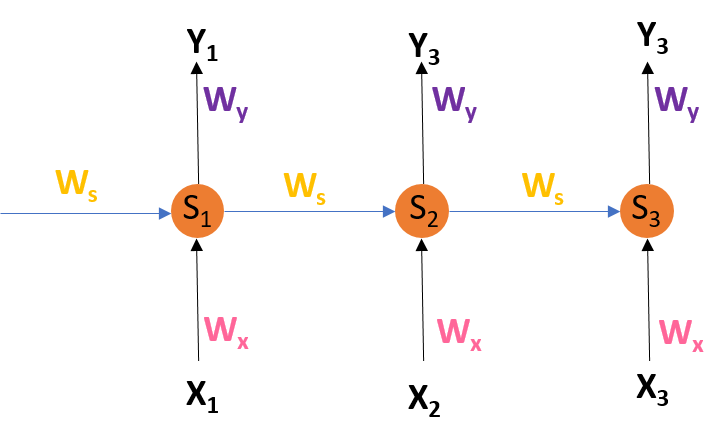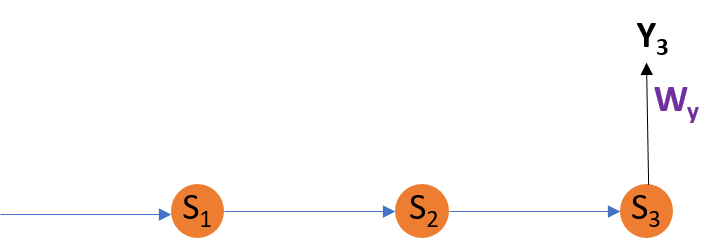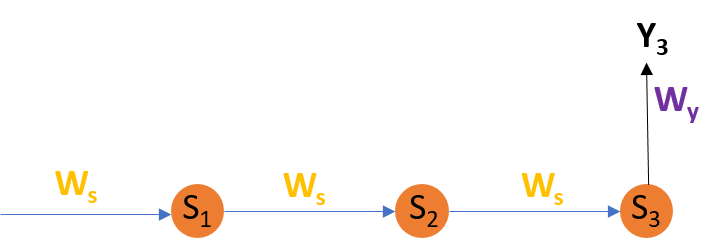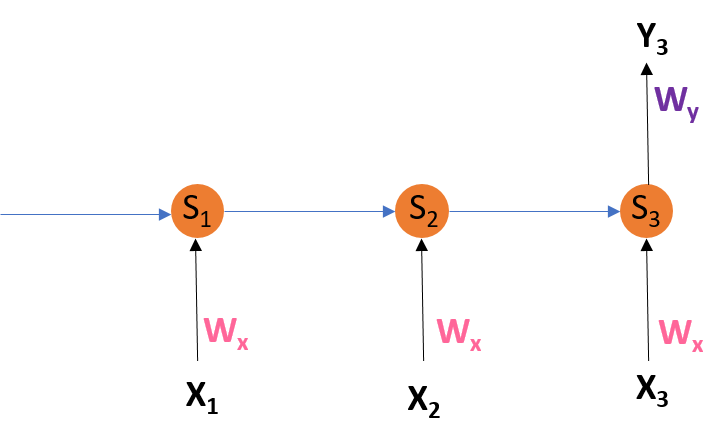# Back Propagation through time – RNN

Introduction:
Recurrent Neural Networks are those networks that deal with sequential data. They predict outputs using not only the current inputs but also by taking into consideration those that occurred before it. In other words, the current output depends on current output as well as a memory element (which takes into account the past inputs).
For training such networks, we use good old backpropagation but with a slight twist. We don’t independently train the system at a specific time “t”. We train it at a specific time “t” as well as all that has happened before time “t” like t-1, t-2, t-3.

Consider the following representation of a RNN:RNN Architecture

S1, S2, S3 are the hidden states or memory units at time t1, t2, t3 respectively, and Ws is the weight matrix associated with it.
X1, X2, X3 are the inputs at time t1, t2, t3 respectively, and Wx is the weight matrix associated with it.
Y1, Y2, Y3 are the outputs at time t1, t2, t3 respectively, and Wy is the weight matrix associated with it.
For any time, t, we have the following two equations:where g1 and g2 are activation functions.
Let us now perform back propagation at time t = 3.
Let the error function be:, so at t =3,*We are using the squared error here, where d3 is the desired output at time t = 3.
To perform back propagation, we have to adjust the weights associated with inputs, the memory units and the outputs.
For better understanding, let us consider the following representation:Formula:Explanation:
E3 is a function of Y3. Hence, we differentiate E3 w.r.t Y3.
Y3 is a function of WY. Hence, we differentiate Y3 w.r.t WY.

For better understanding, let us consider the following representation:Formula:Explanation:
E3 is a function of Y3. Hence, we differentiate E3 w.r.t Y3.
Y3 is a function of S3. Hence, we differentiate Y3 w.r.t S3.
S3 is a function of WS. Hence, we differentiate S3 w.r.t WS.
But we can’t stop with this; we also have to take into consideration, the previous time steps. So, we differentiate (partially) the Error function with respect to memory units S2 as well as S1 taking into consideration the weight matrix WS.
We have to keep in mind that a memory unit, say St is a function of its previous memory unit St-1.
Hence, we differentiate S3 with S2 and S2 with S1.
Generally, we can express this formula as:For better understanding, let us consider the following representation:Formula:Explanation:
E3 is a function of Y3. Hence, we differentiate E3 w.r.t Y3.
Y3 is a function of S3. Hence, we differentiate Y3 w.r.t S3.
S3 is a function of WX. Hence, we differentiate S3 w.r.t WX.
Again we can’t stop with this; we also have to take into consideration, the previous time steps. So, we differentiate (partially) the Error function with respect to memory units S2 as well as S1 taking into consideration the weight matrix WX.
Generally, we can express this formula as:Limitations:
This method of Back Propagation through time (BPTT) can be used up to a limited number of time steps like 8 or 10. If we back propagate further, the gradientbecomes too small. This problem is called the “Vanishing gradient” problem. The problem is that the contribution of information decays geometrically over time. So, if the number of time steps is >10 (Let’s say), that information will effectively be discarded.

Going Beyond RNNs:
One of the famous solutions to this problem is by using what is called Long Short-Term Memory (LSTM for short) cells instead of the traditional RNN cells. But there might arise yet another problem here, called the exploding gradient problem, where the gradient grows uncontrollably large.
Solution: A popular method called gradient clipping can be used where in each time step, we can check if the gradient> threshold. If yes, then normalize it.

My Personal Notes arrow_drop_upCheck out this Author's contributed articles.

If you like GeeksforGeeks and would like to contribute, you can also write an article using contribute.geeksforgeeks.org or mail your article to contribute@geeksforgeeks.org. See your article appearing on the GeeksforGeeks main page and help other Geeks.

Please Improve this article if you find anything incorrect by clicking on the "Improve Article" button below.

Improved By : KeshavBalachandar

Article Tags :
Practice Tags :

5

Please write to us at contribute@geeksforgeeks.org to report any issue with the above content.